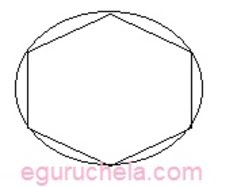# Regular Polygon Calculator

Calculate the Area of the Polygon, Area of the circle, Area Ratio and Side Length for given circumradius and number of sides.

 Enter the number of sides of the polygon(minimum 3) : Enter the circumradius :

 Length of the side of the polygon : Area of the Polygon : Area of the circle : Area Ratio :